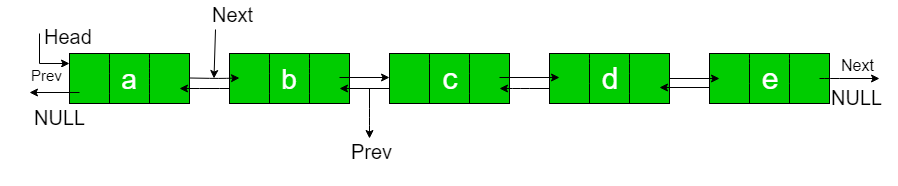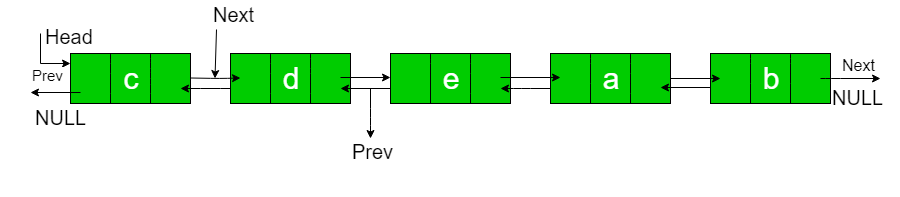# Java Program to Rotate Doubly linked list by N nodes

Given a doubly linked list, rotate the linked list counter-clockwise by N nodes. Here N is a given positive integer and is smaller than the count of nodes in linked list.N = 2
Rotated List:Examples:

```Input : a  b  c  d  e   N = 2
Output : c  d  e  a  b

Input : a  b  c  d  e  f  g  h   N = 4
Output : e  f  g  h  a  b  c  d ```

To rotate the Doubly linked list, we need to change next of Nth node to NULL, next of last node to previous head node, and prev of head node to last node and finally change head to (N+1)th node and prev of new head node to NULL (Prev of Head node in doubly linked list is NULL)
So we need to get hold of three nodes: Nth node, (N+1)th node and last node. Traverse the list from beginning and stop at Nth node. Store pointer to Nth node. We can get (N+1)th node using NthNode->next. Keep traversing till end and store pointer to last node also. Finally, change pointers as stated above and at Last Print Rotated List using
PrintList Function.

## Java

 `// Java program to rotate a Doubly linked ` `// list counter clock wise by N times ` `class` `GfG { `   `/* Link list node */` `static` `class` `Node` `{ ` `    ``char` `data; ` `    ``Node prev; ` `    ``Node next; ` `}` `static` `Node head = ``null``;`   `// This function rotates a doubly linked ` `// list counter-clockwise and updates the ` `// head. The function assumes that N is ` `// smallerthan size of linked list. It ` `// doesn't modify the list if N is greater ` `// than or equal to size ` `static` `void` `rotate( ``int` `N) ` `{ ` `    ``if` `(N == ``0``) ` `        ``return``; `   `    ``// Let us understand the below code ` `    ``// for example N = 2 and ` `    ``// list = a <-> b <-> c <-> d <-> e. ` `    ``Node current = head; `   `    ``// current will either point to Nth ` `    ``// or NULL after this loop. Current ` `    ``// will point to node 'b' in the ` `    ``// above example ` `    ``int` `count = ``1``; ` `    ``while` `(count < N && current != ``null``)` `    ``{ ` `        ``current = current.next; ` `        ``count++; ` `    ``} `   `    ``// If current is NULL, N is greater ` `    ``// than or equal to count of nodes ` `    ``// in linked list. Don't change the ` `    ``// list in this case ` `    ``if` `(current == ``null``) ` `        ``return``; `   `    ``// current points to Nth node. Store ` `    ``// it in a variable. NthNode points to ` `    ``// node 'b' in the above example ` `    ``Node NthNode = current; `   `    ``// current will point to last node ` `    ``// after this loop current will point ` `    ``// to node 'e' in the above example ` `    ``while` `(current.next != ``null``) ` `        ``current = current.next; `   `    ``// Change next of last node to previous ` `    ``// head. Next of 'e' is now changed to ` `    ``// node 'a' ` `    ``current.next = head; `   `    ``// Change prev of Head node to current ` `    ``// Prev of 'a' is now changed to node 'e' ` `    ``(head).prev = current; `   `    ``// Change head to (N+1)th node ` `    ``// head is now changed to node 'c' ` `    ``head = NthNode.next; `   `    ``// Change prev of New Head node to NULL ` `    ``// Because Prev of Head Node in Doubly ` `    ``// linked list is NULL ` `    ``(head).prev = ``null``; `   `    ``// change next of Nth node to NULL ` `    ``// next of 'b' is now NULL ` `    ``NthNode.next = ``null``; ` `} `   `// Function to insert a node at the ` `// beginning of the Doubly Linked List ` `static` `void` `push(``char` `new_data) ` `{ ` `    ``Node new_node = ``new` `Node(); ` `    ``new_node.data = new_data; ` `    ``new_node.prev = ``null``; ` `    ``new_node.next = (head); ` `    ``if` `((head) != ``null``) ` `        ``(head).prev = new_node; ` `head = new_node; ` `} `   `/* Function to print linked list */` `static` `void` `printList(Node node) ` `{ ` `    ``while` `(node != ``null` `&& node.next != ``null``)` `    ``{ ` `        ``System.out.print(node.data + ``" "``); ` `        ``node = node.next; ` `    ``} ` `    ``if``(node != ``null``)` `    ``System.out.print(node.data); ` `} `   `// Driver's Code ` `public` `static` `void` `main(String[] args) ` `{ ` `    ``/* Start with the empty list */` `    ``// Node head = null; `   `    ``/* Let us create the doubly ` `    ``linked list a<->b<->c<->d<->e */` `    ``push( ``'e'``); ` `    ``push( ``'d'``); ` `    ``push(``'c'``); ` `    ``push(``'b'``); ` `    ``push( ``'a'``); `   `    ``int` `N = ``2``; `   `    ``System.out.println(``"Given linked list "``); ` `    ``printList(head); ` `    ``rotate( N); ` `    ``System.out.println();` `    ``System.out.println(``"Rotated Linked list "``); ` `    ``printList(head); ` `} ` `} `   `// This code is contributed by Prerna Saini`

Output:

```Given linked list
a  b  c  d  e